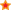for Secondary Education in the UK.Find Resources By Subjects
Information

# Numeracy in Year 7: Three Part Lessons

Price: £25.00
Age Range: KS3

A comprehensive resource for the National Numeracy Strategy. Each lesson plan follows the three-part lesson idea with detailed guidance for teachers.

Topics include: Using and Applying Mathematics to Solve Problems; Calculations Using Written and Calculator Methods; Number Operations and the Relationship Between Them; Integers; Powers; Roots; Place Value; Ordering; Rounding; Fractions; Decimals; Percentage; Ratio; Proportion; Sequences; Functions; Graphs; Equations; Formulae; Shape; Space; Measures.

## Buy this publication:

Quantity Format
Size: 73 Pages
ISBN: 978 1 86025 367 6
Author(s): David Knock
Code: NIY7
Popularity rank: 172
Average Customer Review:based on 1 review

# Contents List

#### LESSONS

• 7 JUST CHECKING Calculations: written methods. Calculations: checking results.
• 9 A PROBLEM SOLVED Using and applying mathematics to solve problems: applying mathematics and solving problems. Calculations: calculator methods. Calculations: checking results.
• 11 THE BIG FREEZE Number and the number system: integers, powers and roots.
• 13 WHATEVER NEXT Algebra: sequences, functions and graphs.
• 15 INTO THE UNKNOWN Algebra: equations, formulae and identities.
• 17 RULES AND MORE RULES Algebra: sequences, functions and graphs.
• 19 SPREAD THE RULE Algebra: sequences, functions and graphs.
• 21 A FAIR SWAP Algebra: equations, formulae and identities.
• 23 GO WITH THE FLOW Algebra: sequences, functions and graphs.
• 25 A PRODUCT OF OUR TIMES Calculations: written methods.
• 27 FAIR SHARES Calculations: written methods.
• 29 DECI TIMES Numbers and the number system: place value, ordering and rounding.
• 31 DECI SHARES Numbers and the number system: place value, ordering and rounding.
• 33 SO NEAR AND NOT TOO FAR Numbers and the number system: place value, ordering and rounding.
• 35 THE ROOT TO ALL SQUARES Numbers and the number system: integers, powers and roots.
• 37 TO ADD TO OR TAKE AWAY Numbers and the number system: place value, ordering and rounding.
• 39 A LITTLE BIT MORE, A LITTLE BIT LESS Calculations: number operations and the relationship between them.
• 41 IN TOTAL Calculations: written methods.
• 43 A LITTLE BIT LESS Calculations: written methods.
• 45 WHAT ARE THE POSSIBILITIES? Calculations: calculator methods.
• 47 A FRACTION MORE Numbers and the number system: fractions, decimals, percentages, ratio and proportion.
• 49 ARE YOU CERTAIN? Numbers and the number system: fractions, decimals, percentages, ratio and proportion.
• 51 TOTAL FRACTIONS Numbers and the number system: fractions, decimals, percentages, ratio and proportion.
• 53 LOOKS DIFFERENT... SAME VALUE Numbers and the number system: fractions, decimals, percentages, ratio and proportion.
• 55 SHARE AND SHARE ALIKE Numbers and the number system: fractions, decimals, ratio and proportion.
• 57 ARE YOU 100%? Numbers and the number system: fractions, decimals, ratio and proportion.
• 59 KNOW YOUR CALCULATOR Calculations: number operations and the relationship between them Calculations: calculator methods.
• 61 MADE TO MEASURE Shape, space and measures: measures and mensuration.
• 62 ANSWERS Answers to the exercises on the student pages.
• 69 APPENDIX Web page exercises and answers.

## Reviews

04/09/07

### Simon Davis at n/a

Am really impressed so far. Working well with kids whose attention span is somewhat limited! Clear lesson plans and excellent resources that take a bit of the sting out of planning leaving me more time to access myspace during PPA sessions!